# Inner product

In mathematics, an inner product is an abstract notion on general vector spaces that is a generalization of the concept of the dot product in the Euclidean spaces. Among other things, the inner product on a vector space makes it possible to define the geometric operation of projection onto a complete subspace (in the metric topology induced by the inner product), just like how the dot product makes it possible to define, in the Euclidean spaces, the projection of a vector onto the subspace spanned by a set of other vectors. The projection operation is a powerful geometric tool that makes the inner product a desirable convenience, especially for the purposes of optimization and approximation.

## Formal definition of inner product

Let X be a vector space over a field 𝔽. In applications outside pure mathematics the field 𝔽 is usually the field of complex numbers ℂ or its subfield of real numbers ℝ. The complex conjugate of a complex number c ∈ ℂ is indicated by . When a number c is real, its imaginary part is zero and = c. Conversely, if = c then c is real.

An inner product ⟨ ⋅ , ⋅ ⟩ on X is a map from the Cartesian product X×X to 𝔽 with the following properties:

1.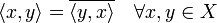$\langle x,y\rangle=\overline{\langle y,x\rangle}\quad \forall x,y \in X$ (in particular it means that ⟨ x, x ⟩ is real)

2.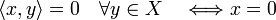$\langle x,y\rangle=0\quad \forall y \in X\quad \Longleftrightarrow x=0$ (inner product is non-degenerate).

3.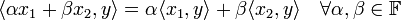$\langle \alpha x_1+\beta x_2,y\rangle= \alpha \langle x_1, y\rangle+\beta \langle x_2, y\rangle \quad \forall \alpha,\beta \in \mathbb{F}$ and$\forall x_1,x_2,y \in X$ (linear in the first slot)

4.$\langle x,\alpha y_1+\beta y_2 \rangle= \bar\alpha \langle x, y_1\rangle +\bar\beta \langle x, y_2\rangle \quad \forall \alpha,\beta \in \mathbb{F}$ and$\forall x,y_1,y_2 \in X$ (anti-linear in the second slot)

5.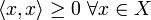$\langle x,x\rangle \geq 0\,\, \forall x \in X$

6.$\langle x,x\rangle=0 \Longleftrightarrow x=0$

Properties 1 and 2 imply that if ⟨ x, y ⟩ = 0 ∀ xX (x in the first slot) that y = 0 (The inner product is non-degenerate in both slots).

Properties 3 and 4 are called sesquilinear. Note that some authors, especially those working in quantum mechanics, may define an inner product to be anti-linear in the first slot and linear in the second slot, this is just a matter of preference.

Properties 5 and 6 define the inner product to be positive definite. Vector spaces with positive definite inner product are sometimes called Euclidean. Some authors distinguish proper and improper Euclidean spaces. The former have a positive definite inner product and the latter have an indefinite, non-degenerate, inner product (lack property 5 and 6). An example of an improper Euclidean space is Minkowski space.

## Norm and topology induced by an inner product

The positive definite inner product induces a norm$\|\cdot\|$ on X defined by |x|= ⟨ x,x½ ≥ 0. Therefore it also induces a metric topology on X via the metric d(x,y)= |xy|. Note that d(x,y) = 0 if and only if x = y.

## Inner product on a finite-dimensional space

Consider a n-dimensional complex vector space V with a non-degenerate inner product ⟨ ⋅, ⋅⟩ that is not necessarily definite (properties 5 and 6 are not stipulated). However, the inner product is conjugate-symmetric and non-degenerate (properties 1 and 2 above do hold, as do properties 3 and 4). Often inner products are required to be positive definite, but since this excludes the Minkowksi metric, which plays an important role in special theory of relativity, this will not be required in this section.

Given a basis ei the matrix g with elements gij = ei , ej is the metric tensor (also known as fundamental tensor) relative to the given basis. It will be shown that g can brought into the following form by a suitable choice of a basis of V: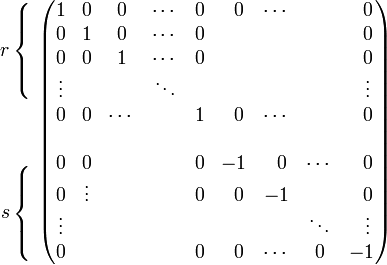\begin{align} r\left\{ \begin{matrix}\; \\ \; \\ \; \\ \; \\ \end{matrix}\right. \\ \\ \\ s\left\{ \begin{matrix}\; \\ \; \\ \; \\ \; \\ \end{matrix}\right. \\ \end{align} \begin{pmatrix} 1 & 0 & 0 &\cdots & 0 & \;\;0 & \cdots & & \;\;0 \\ 0 & 1 & 0 &\cdots & 0 & & & & \;\;0 \\ 0 & 0 & 1 &\cdots & 0 & & & & \;\;0 \\ \vdots & & &\ddots & & & & &\;\;\vdots \\ 0 & 0 & \cdots & & 1 & \;\;0 & \cdots & & \;\;0 \\ & & & & & & & & \\ 0 & 0 & & & 0 & -1 & \;\;0 & \cdots & \;\;0 \\ 0 & \vdots & & & 0 & \;\;0& -1 & & \;\;0 \\ \vdots & & & & & & & \ddots &\;\;\vdots \\ 0 & & & & 0 & \;\;0& \cdots & 0 & -1 \\ \end{pmatrix}$\equiv \operatorname{diag}(\underbrace{1, 1, \cdots, 1}_{r}, \underbrace{-1, -1, \cdots, -1}_{s})$

where n = r+s. The pair (r, s) is the signature of the inner product.

In this section the inner product will be taken to be conjugate-symmetric in the first slot: c x, y = x, y for complex number c. This will facilitate the notation.

The matrix g represents a second rank covariant tensor. That is, given a basis transformation with matrix B,$g'_{kl} = \sum_{i=1}^n \sum_{j=1}^n \bar{B}_{ik}B_{jl} \langle e_{i},\; e_{j} \rangle= \sum_{i=1}^n \sum_{j=1}^n \bar{B}_{ik}B_{jl} \;g_{ij}.$

As an n×n matrix g is Hermitian and non-singular. If g were singular there would be a non-zero n-tuple of complex numbers (column vector) k in its kernel, and for an arbitrary n-tuple it would follow that,$\mathbf{g} \mathbf{k} = \mathbf{0} \rightarrow \boldsymbol{\ell}^\dagger \mathbf{g} \mathbf{k} = 0 \Longleftrightarrow \sum_{ij} \bar{\ell}_i \langle e_i,\; e_j\rangle k_j = \langle\ell, k \rangle = 0$

with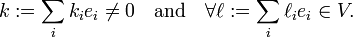$k := \sum_i k_i e_i \ne 0\quad \hbox{and}\quad \forall \ell := \sum_i \ell_i e_i \in V.$

The requirement of non-degeneracy of inner product gives that vector k = 0, and then also its component vector k is zero, so there is a contradiction: g is non-singular.

As an n×n Hermitian matrix, g can be unitarily diagonalized and has real eigenvalues. That is, there is an n×n unitary matrix U, such that$\mathbf{U}^\dagger \mathbf{g} \mathbf{U} = \operatorname{diag}(\alpha_1, \alpha_2, \ldots, \alpha_n),\qquad \alpha_1, \alpha_2, \ldots, \alpha_n \in \mathbb{R}.$

Here U stands for the matrix U in which all elements are transposed and complex-conjugated. (If the inner product is defined to be conjugate-symmetric with respect the second slot, this equation must be suitably modified.) None of the eigenvalues αk is equal to zero. If one or more eigenvalues were zero the determinant det(g) = 0 and g is singular (a square matrix is singular if and only if its determinant vanishes), in contradiction to its non-degenerate character. So the following definition of a real diagonal matrix D is possible$\mathbf{D} := \operatorname{diag}( \frac{1}{\sqrt{|\alpha_1|}}, \frac{1}{\sqrt{|\alpha_2|}},\cdots, \frac{1}{\sqrt{|\alpha_n|}} ).$

Multiply the one but last equation on the left by DT = D = D and on the right by D,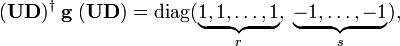$(\mathbf{UD})^\dagger\; \mathbf{g}\; (\mathbf{UD}) = \operatorname{diag}(\underbrace{1, 1, \ldots,1}_r,\; \underbrace{-1, \ldots,-1}_s),$

where it is used that$\frac{\alpha_i}{\sqrt{|\alpha_i|}\;\sqrt{|\alpha_i|}} = \begin{cases} \;\;1 &\; \hbox{if}\; \alpha_i > 0 \\ -1 &\; \hbox{if}\; \alpha_i < 0 \end{cases}$

and it is assumed that the columns of the eigenvector matrix U are arranged such that the r positive eigenvalues precede the s negative eigenvalues.

Summarizing, there exist a basis oi for V, defined by$o_i := \sum^n_{i=1} e_j\; (UD)_{ji}$

with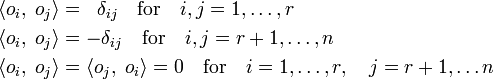\begin{align} \langle o_i,\;o_j\rangle &=\;\; \delta_{ij}\quad \text{for}\quad i,j=1,\ldots, r\\ \langle o_i,\;o_j \rangle &= -\delta_{ij}\quad\text{for}\quad i,j=r+1,\ldots, n \\ \langle o_i,\;o_j \rangle &= \langle o_j,\;o_i \rangle = 0 \quad\text{for}\quad i=1, \ldots, r, \quad j= r+1, \ldots n \end{align}

The matrix oi , oj has the shape given at the beginning of this section. This basis oi is orthonormal with respect to the given inner product. When s = 0 (all r = n eigenvalues positive) the inner product is positive-definite and V is a proper Euclidean space. When s = 1 and r = 3 (or equivalently s = 3, r = 1), and the ground field of the space is ℝ ⊂ ℂ, the 4-dimensional space is a Minkowski space. Such a space is referred to as an improper Euclidean or pseudo-Euclidean space.

### Example

The simplest example of an indefinite metric tensor g is on real 2-dimensional space,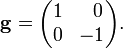$\mathbf{g} = \begin{pmatrix} 1 & \;\;0 \\ 0 & -1 \\ \end{pmatrix}.$

A non-zero vector (x1, x2) with zero length squared is obtained from$|\mathbf{x}|^2 = (x_1, x_2)\; \mathbf{g}\; \begin{pmatrix} x_1\\x_2\end{pmatrix} = 0\quad \Longrightarrow \quad x_1^2 - x_2^2 = 0.$

Hence there are two solutions x1,2$\mathbf{x}_{1,2} = \lambda \begin{pmatrix}\;\;1 \\ \pm 1 \end{pmatrix}, \quad \lambda \in \mathbb{R}.$

In the theory of special relativity x2 = c t, where c is the speed of light and t the time, while x1 is the space-coordinate of an object. The zero-length vector satisfies$c^2 = \left(\frac{x_1}{t}\right)^2$

Hence x1/t is the velocity of an object that moves with the speed of light.

## Reference

1. T. Kato, A Short Introduction to Perturbation Theory for Linear Operators, Springer-Verlag, New York (1982), ISBN 0-387-90666-5 p. 49Some content on this page may previously have appeared on Citizendium.